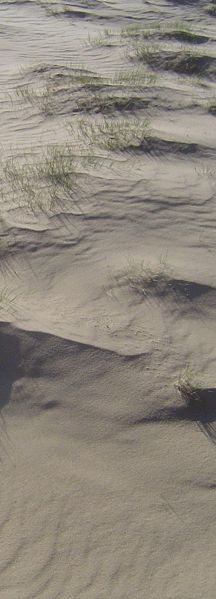# Tutorials¶Here are the many tutorials I’ve written 1 dealing with various aspects of spatial statistics. Please note the dates of each one, the older ones may be partly obsolete or require some adjustment to new versions of the computer programs.

Many of the documents are provided as R Markdown sources. These are most easily processed in the R Studio environment.

## Geostatistics¶

Spatial analysis in R is transitioning to the “Simple Features” representation of spatial objects, as implemented in the sf package. Many of the tutorials listed here were developed with the earlier “Spatial Objects in R” representation, as implemented in the `sp` package. Be alert also to changes in the GDAL and PROJ packages when specifying or transforming coördinate reference systems.

• Spatial simulated annealing

Step-by-step explanation of a method to optimally place sample points in order to minimize the maximum or average kriging variance. This is especially useful in irregularly-shaped areas or areas with existing samples.

• Distance education course Geostatistics & Open-source statistical computing

• Supplementary exercises

• Exercise: Change of support

All geographical measurements are made on some support, that is, an interval (1-D), area (2-D) or volume (3-D) of some finite size. As long as the measurements, interpretations, and predictions all refer to the same support, techniques that treat the support as a 0-D point are satisfactory. But if measurements and predictions are made on different supports, the relation between them must be determined and used to adjust the geostatistical analysis.

• Exercise: Compositional variables

Certain (geo)statistical variables, when considered as a group, are not independent in feature space, because they are constrained to sum to some constant; the set of these is called a composition. They should not be modelled separately, rather, as a group.

• Exercise: Spatio-temporal Geostatistics

Spatio-temporal observations are those for which both a spatial location (georeference) and a time of observation are recorded, as well as attributes measured at the specified location and time. This exercise introduces space-time geostatistics to analyze attributes in space and time, separately and simultaneously.

• Written by prof.dr.ir. Alfred Stein (University of Twente), formatted and with some more explanation by me. (1) Simulation of spatial correlation in one dimension, (2) Ordinary Kriging, (3) Universal Kriging, (4) Cokriging, (5) Selecting a grid spacing for kriging. Distributed by permission.

### R Markdown tutorials¶

These illustrate some details of geostatistics. Load into R Studio and compile (“knit”) to HTML, or execute chunk-by-chunk

## Spatial analysis¶

Spatial analysis in R is transitioning to the “Simple Features” representation of spatial objects, as implemented in the sf package. Many of the tutorials listed here were developed with the earlier “Spatial Objects in R” representation, as implemented in the `sp` package. Be alert also to changes in the GDAL and PROJ packages when specifying or transforming coördinate reference systems.

• Trend surfaces in R by Ordinary and Generalized Least Squares

A trend surface is a map of some continuous variable, computed as a function of the coördinates. In many cases the assumption that the OLS residuals are spatially-independent is not true, so that GLS must be used to obtain a correct trend-surface formula. This one has been converted to use `sf`.

• Thin-plate spline interpolation with R

Shows how to fit a surface (as in the trend surface) but adjusting to local observations (as in kriging) using 2-D smoothing splines. This is useful if one wants to quickly obtain a clear map showing the main features of the variable, without the model assumptions of trend surfaces or kriging.

• Areal Data and Spatial Autocorrelation R

This tutorial gives an overview of spatial analysis of areal data, that is, attributes of polygonal entities on a map. Typical examples are political divisions, census tracts, and ownership or management parcels. The attribute relates to the whole area of the polygon, and can not be further localized.

• Exercise: Exploratory Data Analysis with GeoDa

GeoDa is an open-source program, cross-platform program designed as a simple tool for exploratory spatial data analysis (ESDA) and some spatial modelling of spatial polygon data, that is, maps of polygon units such as census tracts or political divisions with a set of attributes measured on each one. This uses a portion of the 8-county data of the R exercise (see item just above).

• Point-pattern analysis with R

This tutorial gives an overview of spatial point-pattern analysis. This considers the distribution of one or more sets of points in some bounded region as the result of some stochastic process which produces a finite number of “events” or “occurrences”.

• Optimal partitioning of soil transects with R

Applies the split moving window approach to optimally partition linear series of observations to find “natural” boundaries.

## Spatial sampling¶

• `Spatial Coverage Sampling`

The spcosa R package is described in Walvoort, D. J. J., Brus, D. J., & de Gruijter, J. J. (2010). An R package for spatial coverage sampling and random sampling from compact geographical strata by k-means. Computers & Geosciences, 36(10), 1261–1267. https://doi.org/10.1016/j.cageo.2010.04.005

The aim is to place the sample locations so that they cover the study area as uniformly as possible. In a rectangular area grid sampling will accomplish this, but most study areas are not rectangles. Further, this package accounts for two-stage sampling: we can use the existing points and add new ones in the optimal locations.

1